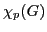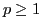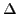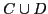# Discrete Algorithms and Computational Complexity

### Friday January 22

9:00-9:30
Pavol Hell (Simon Fraser University, Vancouver)
Generalizations of interval graphs motivated by the constraint satisfaction problem

Recently, interval graphs have naturally arisen in the context of constraint satisfaction problems. Progress in understanding what structures make CSP's tractable suggests generalizations and analogues of interval graphs. I will discuss obstruction characterizations and recognition algorithms for these generalizations. Applications to the CSP dichotomy problem will also be mentioned. This is joint work with Tomas Feder, Jing Huang, and Arash Rafiey.

9:30-10:00
Jaroslav Nesetril (Charles University, Prague)
On effective representation of groups, monoids and posets

We discuss the title of the lecture in the context of graph classes. Some of the results are joint with Jan Hubicka and Patrice Ossona de Mendez.

10:00-10:30
Guillem Perarnau (Universitat Politècnica de Catalunya)
Bounds on the k-th chromatic number of generalized star colorings

A graph has star chromatic number k if it can be colored with k colors in such a way that each color class is a stable set and the subgraph induced by any two color classes is a forest of stars. The star chromatic number can be generalized to,, by requiring that anyclasses induce a graph with treedepth at most i. Nešetřil and Ossona de Mendez proved that, for each,is bounded in the class of bounded expansion graphs. By using the Local Lovász Lemma we give some specific bounds for the subclass of graphs with maximum degree.

11:00-11:30
Jiri Fiala (Charles University, Prague)
Complexity of the distance constrained labeling problem for trees

We show a full complexity dichotomy for the distance constrained labeling problem on the class of trees.

11:30-12:00
Bernard Lidický (Charles University, Prague)
The k-in-a-path problem for claw-free graphs

Testing whether there is an induced path in a graph spanning k given vertices is already NP-complete in general graphs when k=3. We show how to solve this problem in polynomial time on claw-free graphs, when k is not part of the input but an arbitrarily fixed integer. This is a joint work with Jiří Fiala, Marcin Kamiński and Daniël Paulusma.

12:00-12:30
Clemens Huemer (Universitat Politècnica de Catalunya)
On 3-Colorability of Pseudo-Triangulations

Deciding 3-colorability for general plane graphs is known to be an NP-complete problem. However, for certain families of graphs, e.g. triangulations, polynomial time algorithms exist.

We consider the family of pseudo-triangulations, which are a generalization of triangulations, and prove NP-completeness for this class. This also holds, if we bound their face degree to four or consider exclusively pointed pseudo-triangulations with maximum face-degree five. In contrast to these completeness results we show that pointed pseudo-triangulations with maximum face-degree four are always 3-colorable. An according 3-coloring can be found in linear time.

This is a joint work with Oswin Aichholzer, Franz Aurenhammer, Thomas Hackl, Alexander Pilz and Birgit Vogtenhuber.

### Monday January 25

14:00-14:30
Martin Loebl (Charles University, Prague)
Enumeration of perfect matchings

Some recent results will be presented.

14:30-15:00
Rolf Niedermeier (Friedrich-Schiller-Universität, Jena)
Multivariate complexity analysis of some voting problems

We survey some recent results on the parameterized computational complexity of NP-hard problems in the context of voting systems. These problems include Kemeny, Dodgson, and Young voting. Moreover, we consider NP-hard possible winner problems for scoring protocols.

15:00-15:30
Ondra Suchý (Charles University, Prague)
What Makes Equitable Connected Partition Easy

We study the EQUITABLE CONNECTED PARTITION problem: partitioning the vertices of a graph into a specified number of classes, such that each class of the partition induces a connected subgraph, so that the classes have cardinalities that differ by at most one. We examine the problem from the parameterized complexity perspective with respect to various (aggregate) parameterizations involving such secondary measurements as: (1) the number of partition classes, (2) the treewidth, (3) the pathwidth, (4) the minimum size of a feedback vertex set, (5) the minimum size of a vertex cover, (6) and the maximum number of leaves in a spanning tree of the graph. In particular, we show that the problem is W-hard with respect to the first four combined, while it is fixed-parameter tractable with respect to each of the last two alone. The hardness result holds even for planar graphs. The problem is in XP when parameterized by treewidth, by standard dynamic programming techniques. Furthermore, we show that the closely related problem of EQUITABLE COLORING (equitably partitioning the vertices into a specified number of independent sets) is FPT parameterized by the maximum number of leaves in a spanning tree of the graph.

(joint work with Rosa Enciso, Michael R. Fellows, Jiong Guo, Iyad Kanj, and Frances Rosamond)

16:00-16:30
Isolde Adler (Goethe University, Frankfurt am Main)
Making graph minor theory constructive

By the Graph Minor Theorem (previously Wagner's Conjecture), every class of finite graphs that is closed under taking minors has a characterization by *finitely* many excluded minors. Moreover, every minor closed graph class has a cubic time membership test. This membership test uses the excluded minor characterization.

The proof of the Graph Minor Theorem is non-constructive, hence, unfortunately, for many minor closed classes we do not know the corresponding excluded minors! Some work has been done to overcome this non-constructiveness, but still many problems remain unsolved. In this talk we present a method to compute excluded minors from certain given information of a minor ideal. Our method uses graph minor theory, logic and automata. In particular, we show: Given two minor closed classes C and D by their excluded minor characterizations, we can effectively compute an upper bound for the size of the excluded minors of.

This is joint work with Martin Grohe and Stephan Kreutzer

16:30-17:00
Petr Hliněný (Masaryk University, Brno)
Unified Approach to Polynomial Algorithms on Graphs of Bounded (bi-)Rank-width

In this paper we develop new algorithmic machinery for solving hard problems on graphs of bounded rank-width and on digraphs of bounded bi-rank-width in polynomial (XP, to be precise) time. These include, particularly, graph colouring and chromatic polynomial problems, the Hamiltonian path and c-min-leaf outbranching, the directed cut, and more generally MSOL-partitioning problems on digraphs. Our focus on a formally clean and unified approach for the considered algorithmic problems is in contrast with many previous published XP algorithms running on graphs of bounded clique-width, which mostly used ad hoc techniques and ideas. The new contributions include faster algorithms for computing the chromatic number and the chromatic polynomial on graphs of bounded rank-width, and new algorithms for solving the defective colouring, the min-leaf outbranching, and the directed cut problems.

Joint work with Robert Ganian and Jan Obdržálek.

17:00-17:30
Jan Kratochvil (Charles University, Prague)
Testing Planarity of Partially Embedded Graphs

We pose and study the following question: Given a (planar graph) G and a planar embedding of its subgraph H, can this be extended to a noncrossing embedding of the entire graph G? This approach follows the paradigm of completing a partial solution of a particular problem, which has been studied in many different situations before. Unlike in many cases, when the presence of a partial solution in the input makes an otherwise easy problem hard, we show that the planarity question remains polynomial-time solvable. Our algorithm is based on several combinatorial lemmas which show that planarity of partially embedded graphs performs the "oncas" behaviour - obvious necessary conditions for planarity are also sufficient. In particular, a 2-connected graph allows an extension of an embedding of its subgraph H if and only the skeleton of each node of its SPQR-tree has an embedding compatible with the given embedding of H. This implies that no dynamic programming is needed for a decision algorithm, the nodes of the SPQR-tree can be processed independently in parallel. It should be noted that though 2-connected graphs form the core situation, nontrivial steps are needed to handle the less connected cases. By refining the techniques and using appropriately adjusted data structures we manage to achieve a linear time algorithm.

On the other hand we consider several generalizations of the problem, e.g. minimizing the number of edges of the partial embedding that need to be rerouted, and argue that they already become NP-hard.

The talk is based on a joint paper with atrizio Angelini, Giuseppe Di Battista, Fabrizio Frati, Vit Jelinek, Maurizio Patrignani, and Ignaz Rutter.